# The Peierls-Nabarro model

The original Peierls–Nabarro (PN) model represents a useful and efficient approach to calculate the core properties of dislocations.

Rudolf Ernst Peierls was a solid state physicist who had only marginal interest in dislocations mostly motivated from discussions and interactions with Egon Orowan.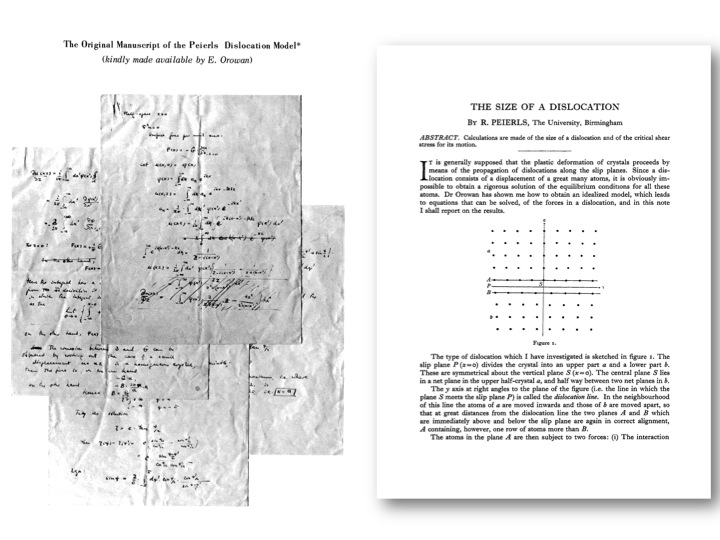"All I did was formulate and solve the equations representing his [Orowan] model. I had wanted this to be a joint paper with Orowan, but he refused

The matter did not seem important at the time and I did not take more interest in the problem of dislocations

At the Batelle symposium (1967) I discovered that this paper had become one of the classics in the field

Nabarro had extended my argument and had also corrected a major algebraic error in my paper"

From Rudolf Peierls "Bird of passage"

The original publication “The size of a dislocation” published in 1940 represents a classics. It has been followed by paper of F.R.N. Nabarro published in 1947: “Dislocations in a simple cubic lattice”. The model is now referred as to “the Peierls-Nabarro (PN) model”. The aim of the PN model is, within a continuum approach, to describe the extension of the core of a dislocation opposed to the singularity that is associated with the Volterra dislocation.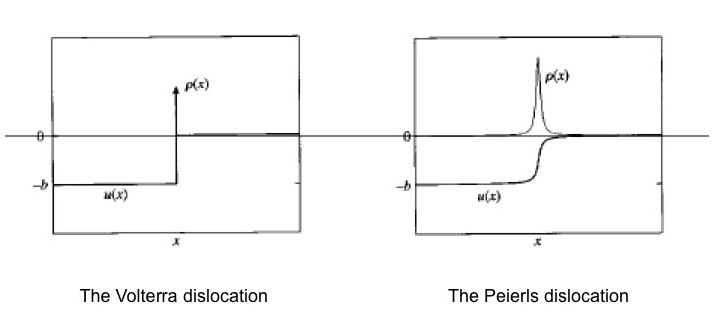The PN model assumes that the misfit region of inelastic displacement is restricted to a single plane where the dislocation will glide. Linear elasticity applies far from it. The dislocation corresponds to a continuous distribution of shear S(x) along the glide plane (x is the coordinate along the displacement direction of the dislocation in the glide plane). S(x) represents the disregistry across the glide plane.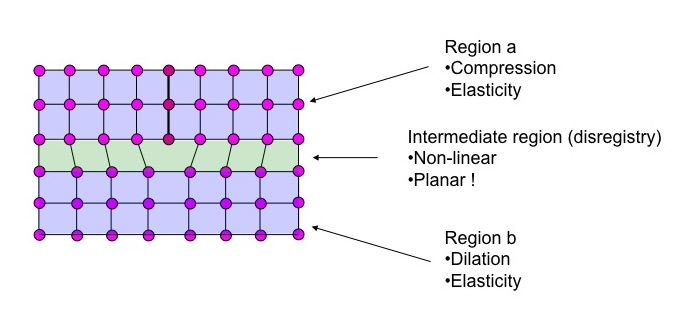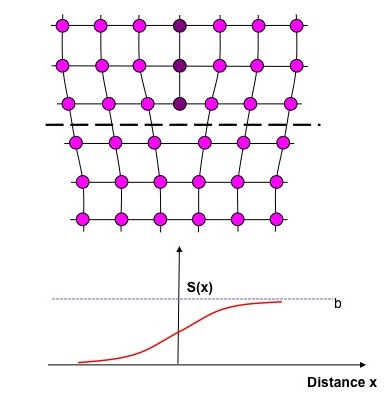The stress generated by such a displacement can be represented by a continuous distribution of infinitesimal dislocations with density ρ(x) for which the total summation is equal to the Burgers vector b. The restoring force, F, acting between atoms on either sides of the interface is balanced by the resultant stress of the distribution leading to the well-known Peierls–Nabarro (PN) equation: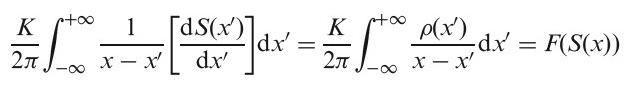where K, the energy coefficient, is a function of the dislocation character. This coefficient can be calculated within the framework of anisotropic elasticity. As originally proposed and further largely developed by Joos and Duesbery (1997), an analytical solution of the PN equation can be found by introducing a sinusoidal restoring force: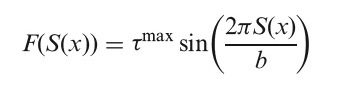where b is the dislocation Burgers vector and τmax is the ideal shear strength (ISS) which is defined as the ‘‘maximum resolved shear stress that an ideal, perfect crystal can suffer without plastically deforming’’. Incorporating this expression in the PN equation leads to a classic solution for the disregistry function: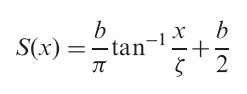where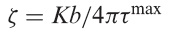represents the half-width of the ρ(x) dislocation distribution.

One can easily check that the disregistry across the glide plane, S(x), verifies the limits, S(x)=0 when x → -∞, and S(x)=b when x → +∞ and the summation of S(x) over the whole space is equal to the Burgers vector b of the dislocation.

In order to obtain the misfit energy corresponding to the Peierls dislocation and to determine the Peierls stress, the sum of the local misfit energy has to be done at the position of atoms rows parallel to the dislocation line. Indeed, the PN equation holds for an elastic continuous medium whereas S(x) can only be defined where an atomic plane is present. The misfit energy can be thus considered as the sum of misfit energies between pairs of atomic planes and can be written as: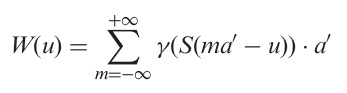where a’ is the periodicity of W, taken as the shortest distance between two equivalent atomic rows in the direction of the dislocation’s displacement. The Peierls stress is then given by: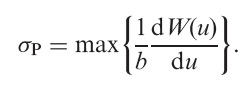The Peierls stress is often described as the critical resolved shear stress (CRSS) at 0K which has les sometimes to some misunderstanding about the relevance of this theoretical concept. As it is described elsewhere, the motion of a dislocation is a stress-assisted thermally activated phenomenon. The evolution of the CRSS with temperature describes the interplay between the influence of stress and temperature with two extreme cases. The Peierls stress at 0K where lattice friction is overcome under the sole influence of stress (without temperature) and the athermal regime where thermal activation frees the dislocation from lattice friction. The Peierls stress can thus be viewed as a mechanical assessment of lattice friction.

In order to solve the misfit energy function analytically, Joos and Duesbery introduced a dimensionless parameter Γ=ζ/a’. Then, some simple formulas can be derived for the extreme cases of very narrow (Γ <<1) and widely spread (Γ >>1) dislocations: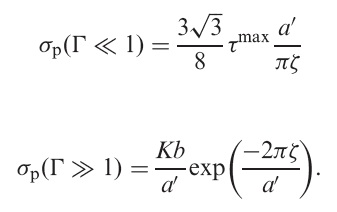The analytical solution presented above can be applied in case of a sinusoidal restoring force and with the knowledge of the ISS value. As demonstrated by Vitek (1968), the restoring force introduced in the PN model is simply the gradient of the so-called generalized stacking fault (GSF):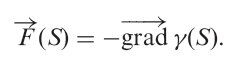The introduction of the GSF energies that can be calculated accurately from the first-principles has renewed interest in the PN model in allowing real dislocations structures to be predicted. The example below where demonstrate in the case of a <100>1 dislocation in SrTiO3 that the PN model is in excellent agreement with experimental HRTEM images.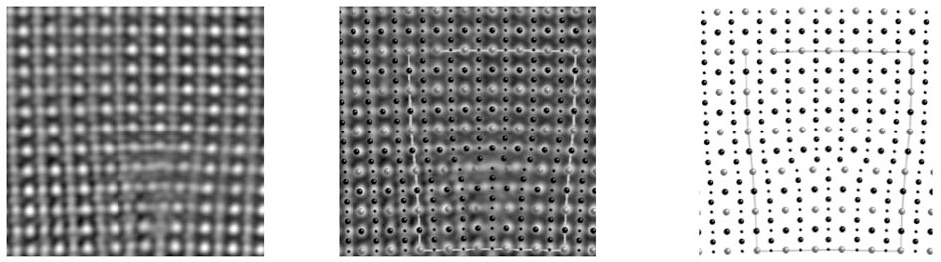Center: comparison of the PN model for a <100>9 dislocation (right) with the experimental TEM micrograph of Jia et al. (2005) reproduced with permission (left).

Despite the high potential of the PN method it is to be remembered that its application is limited by some strong limitations: compact cores of collinear dissociations within a planar core. The examination of GSF provides some good indications as to whether the dislocation core is likely to follow these assumptions or not. When the easiest path for shearing the structure involves overcoming an energy barrier along the Burgers vector (below, left), the core is likely to be simple. An unstable stacking fault indicated by a local minimum of the energy located somewhere on this line would lead to a collinear dissociation. More interesting is the case where such a local minimum does exist but with a location slightly off the Burgers vector. The minimum energy shear path is thus likely to induce a non-collinear dissociation involving this stacking fault. This situation corresponds to the well-known dissociation into Schockley partials in fcc metals.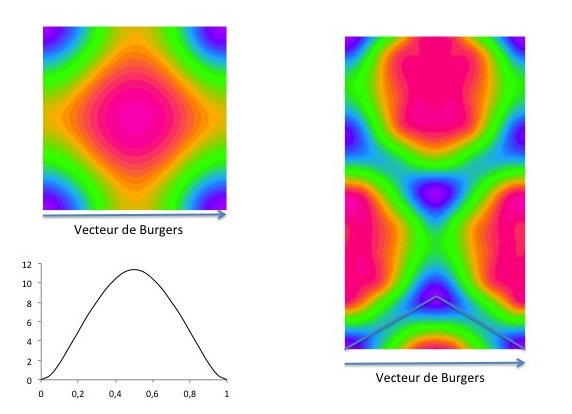It is more difficult to infer in which plane a screw dislocation core would spread and glide since the PN model gives independent solutions for each plane. Several techniques have been proposed to extend the conceptual approach of the PN model to potentially more complex cases. The Peierls–Nabarro–Galerkin method is one of them.

The Peierls–Nabarro–Galerkin (PNG) method is a generalization of the Peierls–Nabarro (PN) method to take into account several glide planes simultaneously and calculate complex (possibly non-collinear and even three-dimensional) cores. As in the initial PN model, the dislocation core structure naturally emerges from the minimizing of an elastic energy (through an approximation of a continuous field representation) and an interplanar potential. The latter is a function of the generalized stacking faults (GSF) energies from which the linear elastic part has been subtracted. In doing so, we strictly comply with the overall linear elastic behaviour expected for small applied strains. In the PNG model two distinct fields are used: u(r), a three-dimensional displacement field of the volume V, and a two-dimensional displacement discontinuity field f(r), which is expressed in the normal basis of the S plane. Thus, u(r) allows one to represent the continuous deformation around the dislocation core, whereas f represents the displacement jump when crossing S.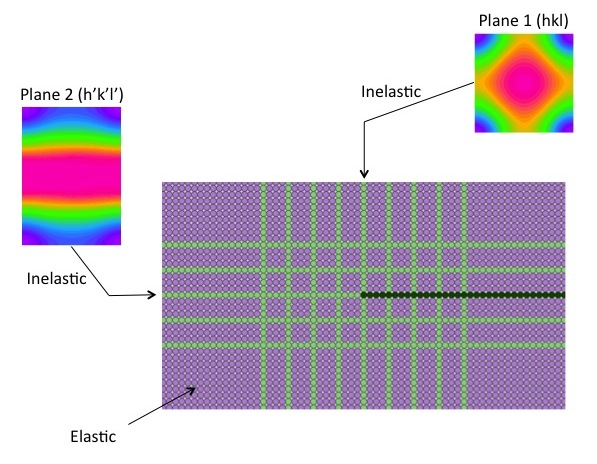Potential glide planes (in green above) are introduced in the surrounding elastic medium (in purple) of the supercell with the same geometry as in the crystal. The dislocation is introduced in the middle of the supercell as a step function (Volterra dislocation). After minimization, the relaxed system displays the shape of the dislocation.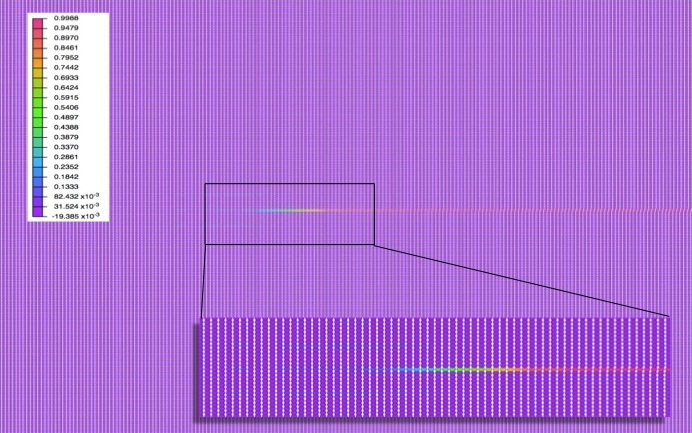In case of a non collinear dissociation, the disregistry exhibits two components. The main one Sx along the Burgers vector and a second one Sy corresponding, for instance, to edge components for a dislocation originally introduced as pure screw (see below).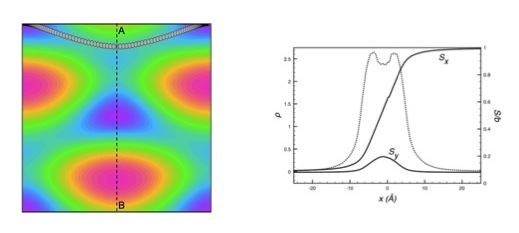References:

D. Ferré, P. Carrez and P. Cordier, Phys. Rev.  B 77, 014106 (2008)

C. L. Jia, A. Thust, and K. Urban, Phys. Rev. Lett. 95, 225506 (2005).

B. Joos and M.S. Duesbery, Phys. Rev. Lett. 78 266 (1997).

F.R.N. Nabarro, Proc. Phys. Soc. 59 256 (1947).

R.E. Peierls, Proc. Phys. Soc. 52 34 (1940).

V. Vitek, Phil. Mag. 18 773 (1968).